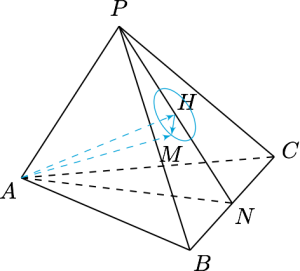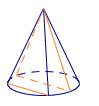# 每日一题空间向量的分解利用图形的对称性，取$BC$的中点$N$，连接$PN,AN$．不难计算得$$AN=PN=8,$$于是三角形$PAN$为正三角形，记$H$为点$A$在平面$PBC$上的投影，则$AH=4\sqrt 3$．因此点$M$的轨迹是半径为$$\sqrt{AM^2-AH^2}=1$$的圆，这样就有$$\cos\alpha=\left|\dfrac{\overrightarrow{AM}\cdot \overrightarrow{BC}}{AM\cdot BC}\right|=\left|\dfrac{\overrightarrow{AH}\cdot \overrightarrow{BC}+\overrightarrow{HM}\cdot \overrightarrow{BC}}{AM\cdot BC}\right|,$$注意到$AH\perp BC$，于是$$\overrightarrow{AH}\cdot\overrightarrow{BC}=0,$$而$\overrightarrow{HM}$与$\overrightarrow{BC}$的夹角取值范围是$[0,\pi]$，因此$\cos\alpha$的最大值为$$\dfrac{HM\cdot BC}{AM\cdot BC}=\dfrac 17.$$

从立体几何角度来看，因为$AM$是母线长为$7$，底面半径为$1$的圆锥上的任意一条母线，$BC$可以平移到底面上，成为一条弦，要找到一条母线，使得该母线与弦的夹角的最小值．